Function Repository Resource:

# StationaryPoints

Compute the stationary points of a function of one or more variables

Contributed by: Wolfram|Alpha Math Team
 ResourceFunction["StationaryPoints"][expr, {var1,var2, …}] computes the stationary points of expr with respect to the variables vari. ResourceFunction["StationaryPoints"][{expr,domain},{var1,var2,…}] computes the stationary points of expr that lie within the specified domain. ResourceFunction["StationaryPoints"][…, "type"] limits the stationary points returned to those of the given type.

## Details and Options

Allowed values of the optional "type" argument are "Maxima", "Minima", and "SaddlePoints". Using Automatic or omitting the "type" argument returns an Association of stationary points keyed according to their types.
ResourceFunction["StationaryPoints"][expr,{var1,},"type"] returns a list of results, each of the form {valj,{var1sol1j,val2sol2j}}, where the list of rules represents the location of the jth stationary point and valj is the value of expr at that point.
For functions with a repeating pattern of stationary points, ResourceFunction["StationaryPoints"] returns results in terms of one or more undetermined constants ci, which can take any integer value.
In ResourceFunction["StationaryPoints"][{expr,domain},], the argument domain should be a Boolean expression, typically an equality, inequality or logical combination thereof, involving the vari.

## Examples

### Basic Examples (7)

Find the stationary points of a function of one variable:

 In:=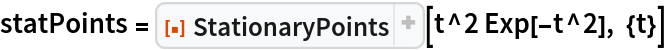Out=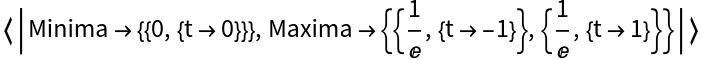Plot the function and its stationary points found above:

 In:=Out=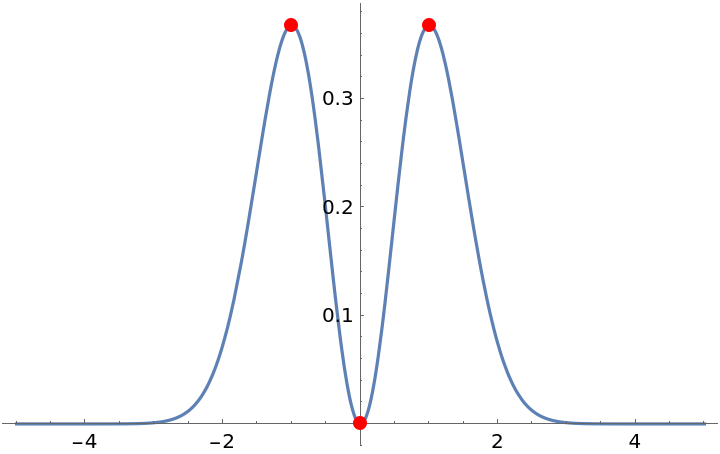Find the stationary points of a periodic function:

 In:=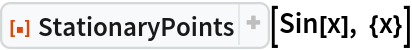Out=In:=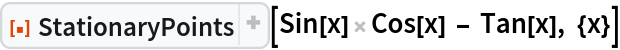Out=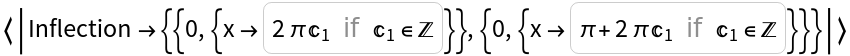Find the stationary points of a function over a restricted domain:

 In:=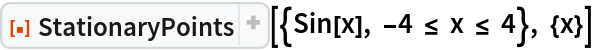Out=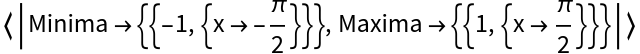In:=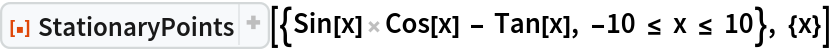Out=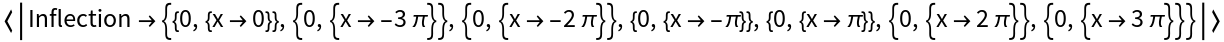Find the stationary points of a function of two variables:

 In:=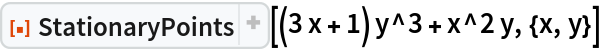Out=Find the stationary points of a function of three variables when restricting to a plane:

 In:=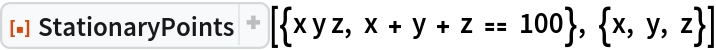Out=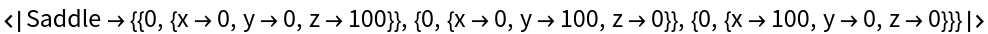Using the "Type" option will return only stationary points of the given type:

 In:=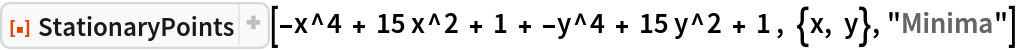Out=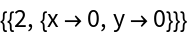In:=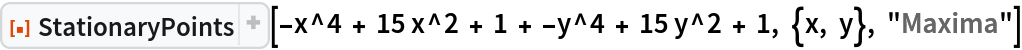Out=In:=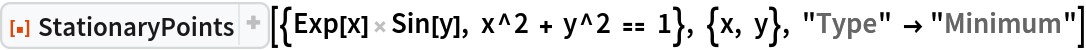Out=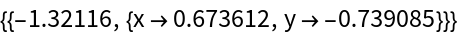Using Automatic as the second argument gives an Association of all stationary points:

 In:=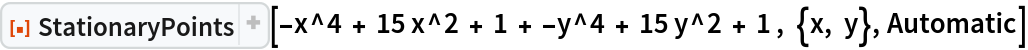Out=### Properties and Relations (2)

StationaryPoints will sometimes return results in terms of Root objects:

 In:=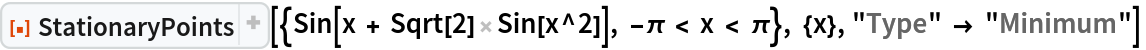Out=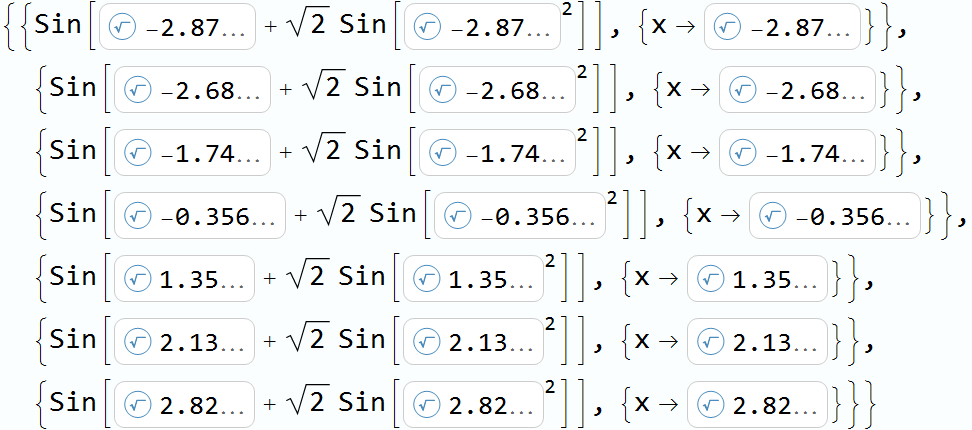Results such as these can be numericized by applying N:

 In:=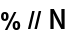Out=For functions with a repeating pattern of stationary points, StationaryPoints returns results in terms of one or more undetermined constants ci, which can take any integer value:

 In:=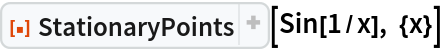Out=### Possible Issues (2)

For functions possessing one or more families of non-isolated stationary points, StationaryPoints may return only the isolated stationary points. For example, the function sin(x3y3) has lines of stationary points along both the x and y axes, as can be seen in the following plot:

 In:=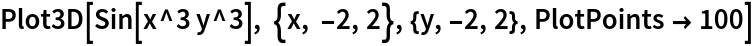Out=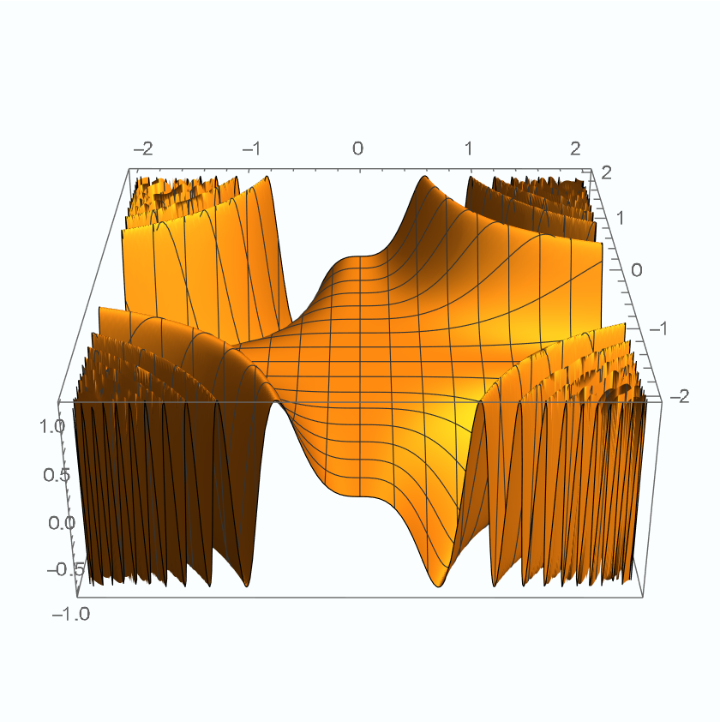These, however, are excluded from the results of StationaryPoints:

 In:=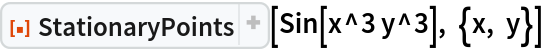Out=## Publisher

Wolfram|Alpha Math Team

## Version History

• 6.0.0 – 23 March 2023
• 5.0.0 – 08 April 2020
• 4.0.0 – 06 September 2019
• 3.0.0 – 12 June 2019
• 2.0.0 – 12 June 2019
• 1.0.0 – 22 February 2019